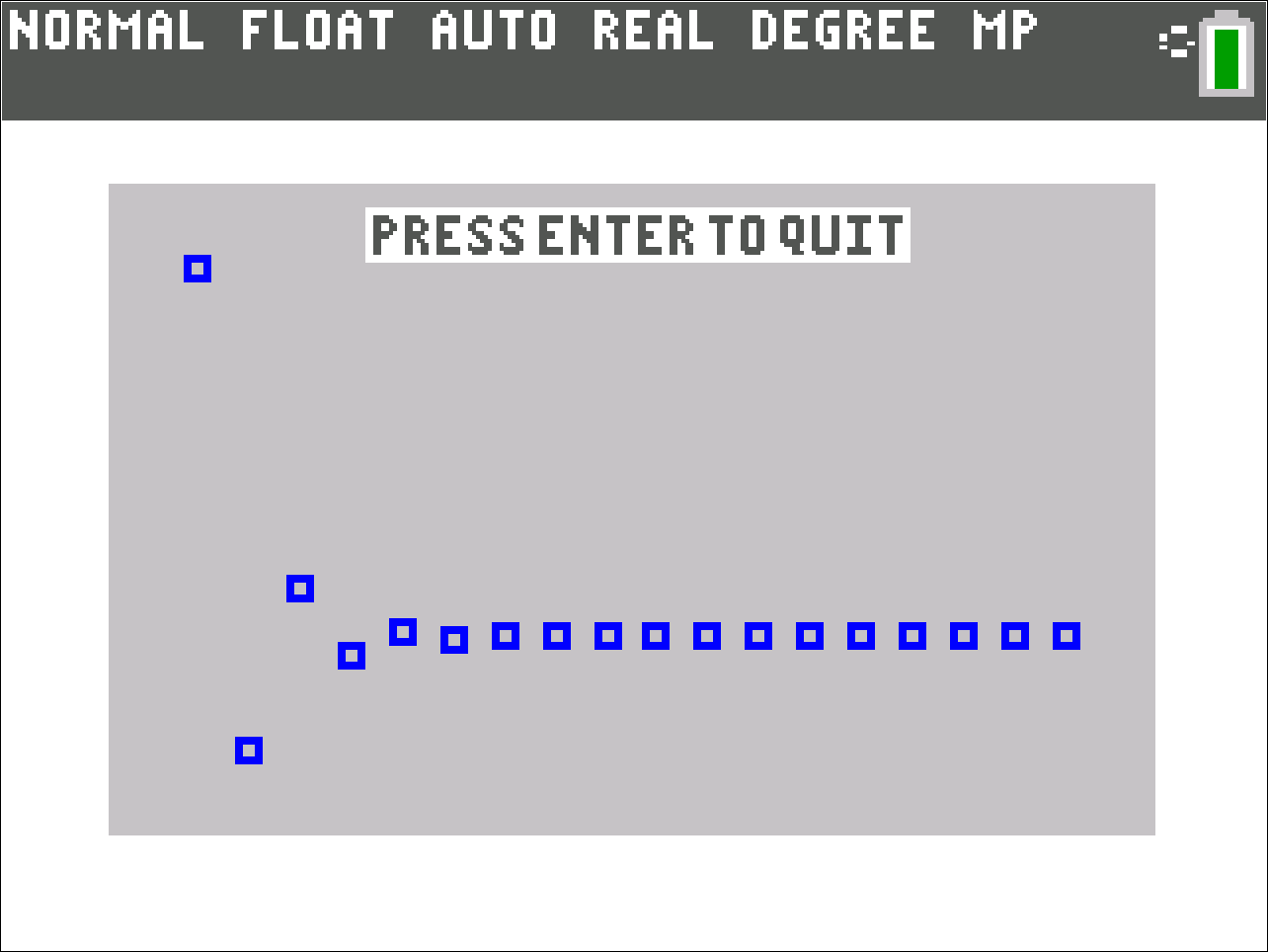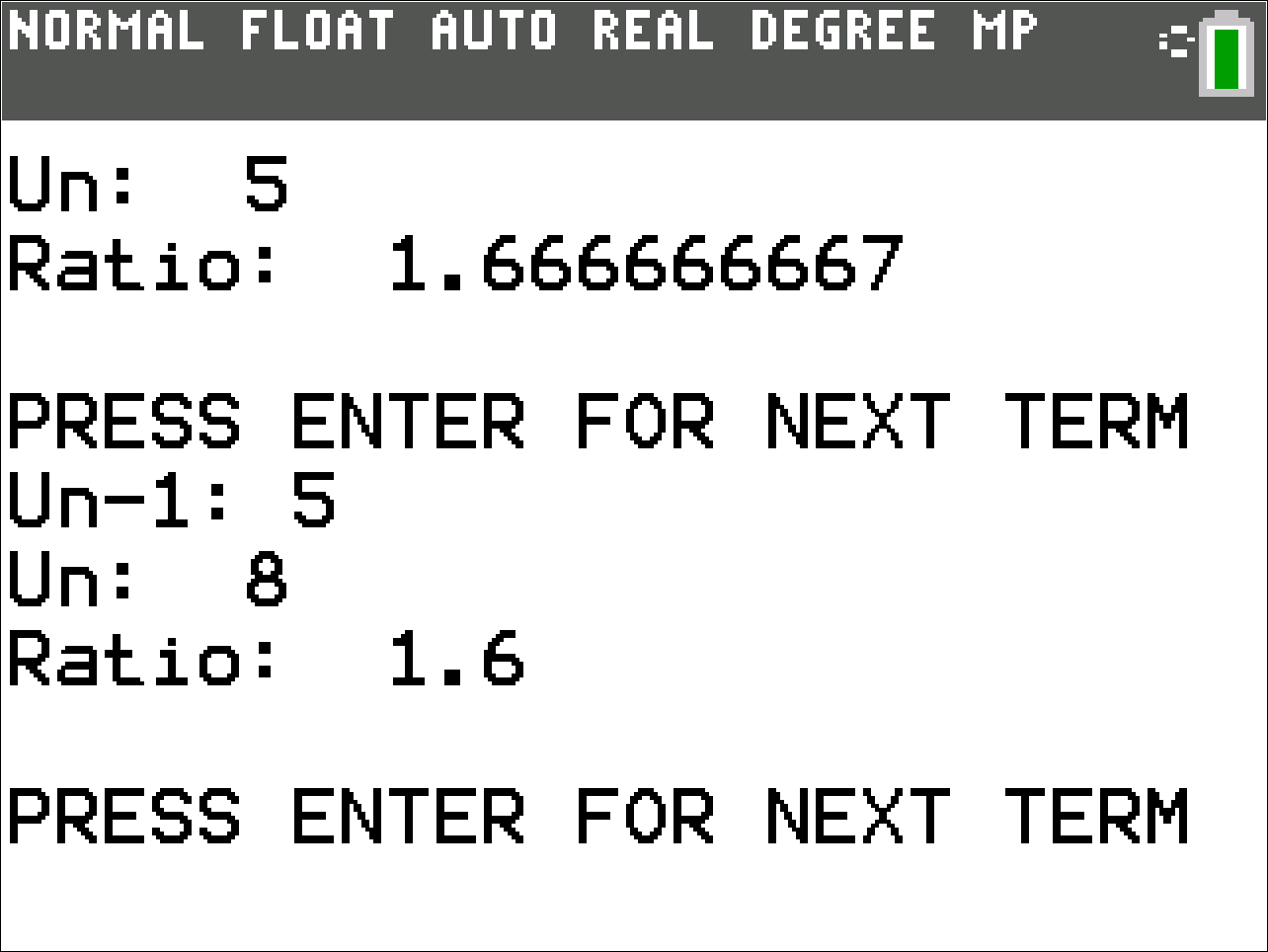Activities

•• Subject Area

• Math: Math General: General

• Author9-12

• Device
• TI-84 Plus
• TI-84 Plus Silver Edition
•TI-84 Plus C Silver Edition
•TI-84 Plus CE
• Report an Issue

Approaching the Golden RatioActivity Overview

Students will observe that the ratio of consecutive terms in all Fibonacci-like sequences approaches the Golden Ratio.

Key Steps

•Students are prompted for the first two terms in their Fibonacci-like sequence where { Un = Un-1 + Un-2}. Then using the number of iterations determined by the students, the program returns the ratio of consecutive terms  (Un / Un-1)for each set of terms in the sequence. Students should explore many different sequences and note that no matter the initial two terms, the ratio of consecutive terms approaches the Golden Ratio.

•Students are challenged to give two initial terms for which the approximation for the Golden Ratio approaches 1.618.... the slowest, and examine the changes in the graph as a result.